# PHYSICS END OF TERM 1 YEAR 10 PROGRESSIVE TEST DECEMBER 2021

Created
Best for live in-class or video conferencing lessonsStart teacher-led lesson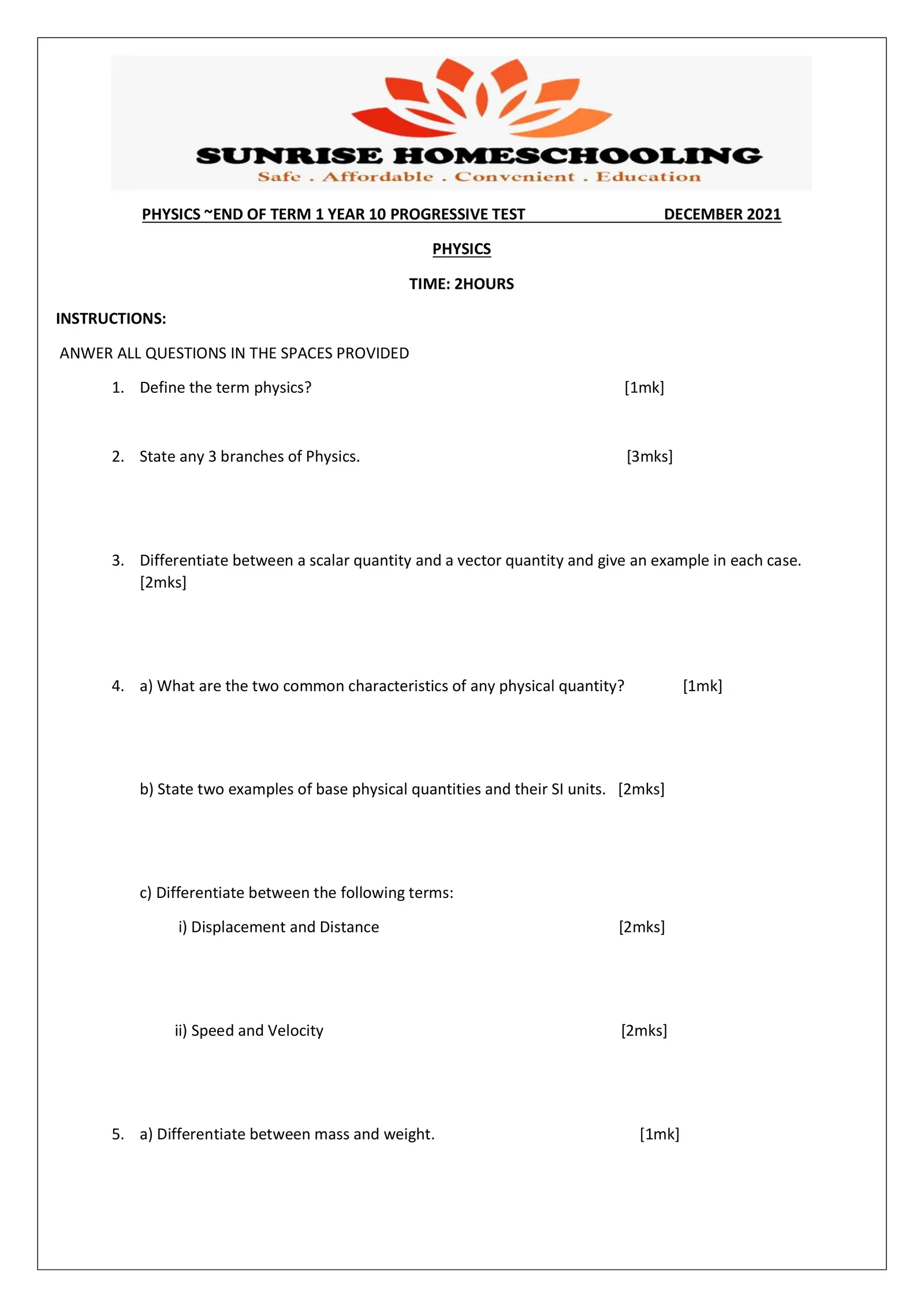PHYSICS ~END OF TERM 1 YEAR 10 PROGRESSIVE TEST DECEMBER 2021 PHYSICS TIME: 2HOURS INSTRUCTIONS: ANWER ALL QUESTIONS IN THE SPACES PROVIDED 1. Define the term physics? [1mk] 2. State any 3 branches of Physics. [3mks] 3. Differentiate between a scalar quantity and a vector quantity and give an example in each case. [2mks] 4. a) What are the two common characteristics of any physical quantity? [1mk] b) State two examples of base physical quantities and their SI units. [2mks] c) Differentiate between the following terms: i) Displacement and Distance [2mks] ii) Speed and Velocity [2mks] 5. a) Differentiate between mass and weight. [1mk]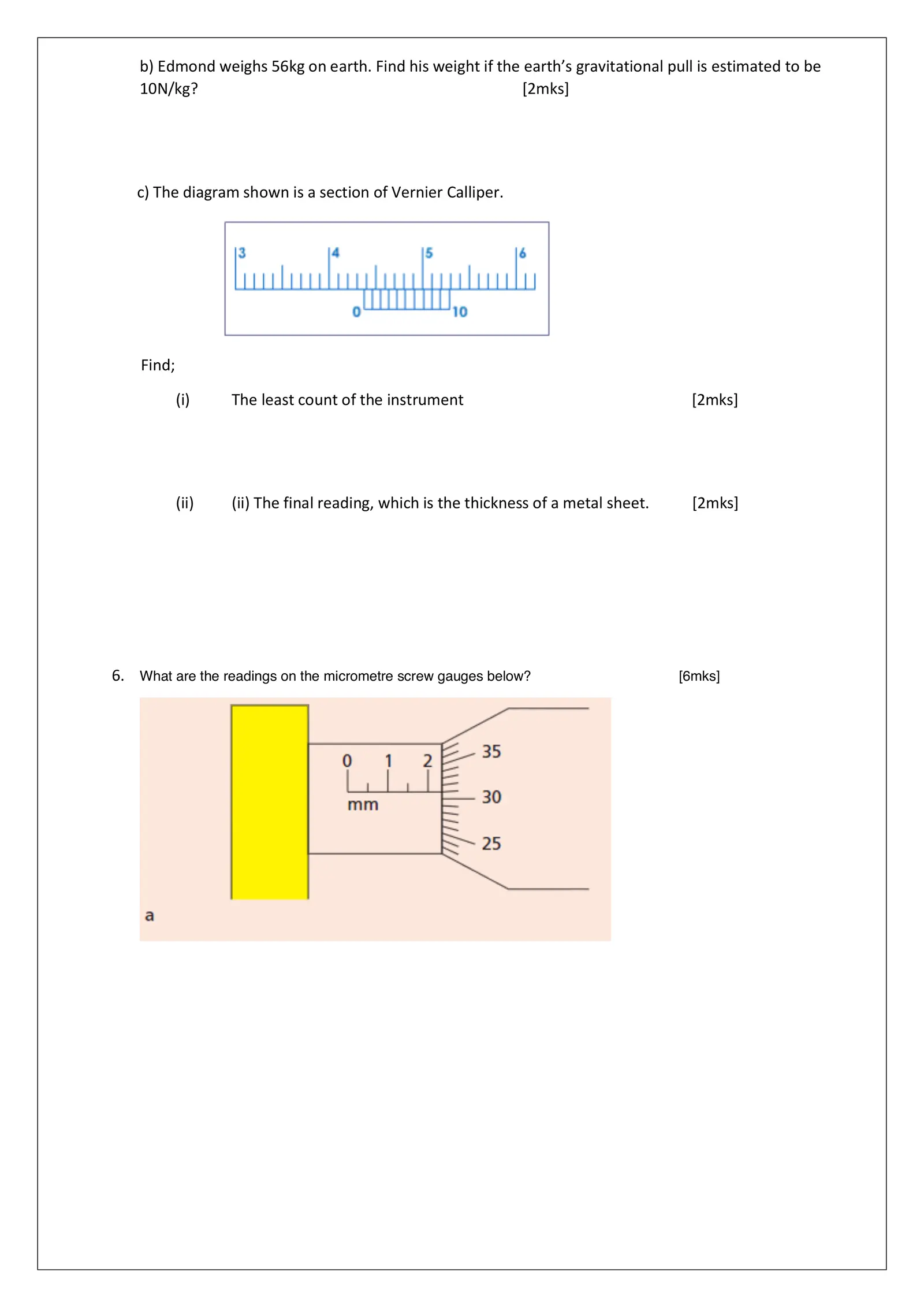b) Edmond weighs 56kg on earth. Find his weight if the earth’s gravitational pull is estimated to be 10N/kg? [2mks] c) The diagram shown is a section of Vernier Calliper. Find; (i) The least count of the instrument [2mks] (ii) (ii) The final reading, which is the thickness of a metal sheet. [2mks] 6. What are the readings on the micrometre screw gauges below? [6mks]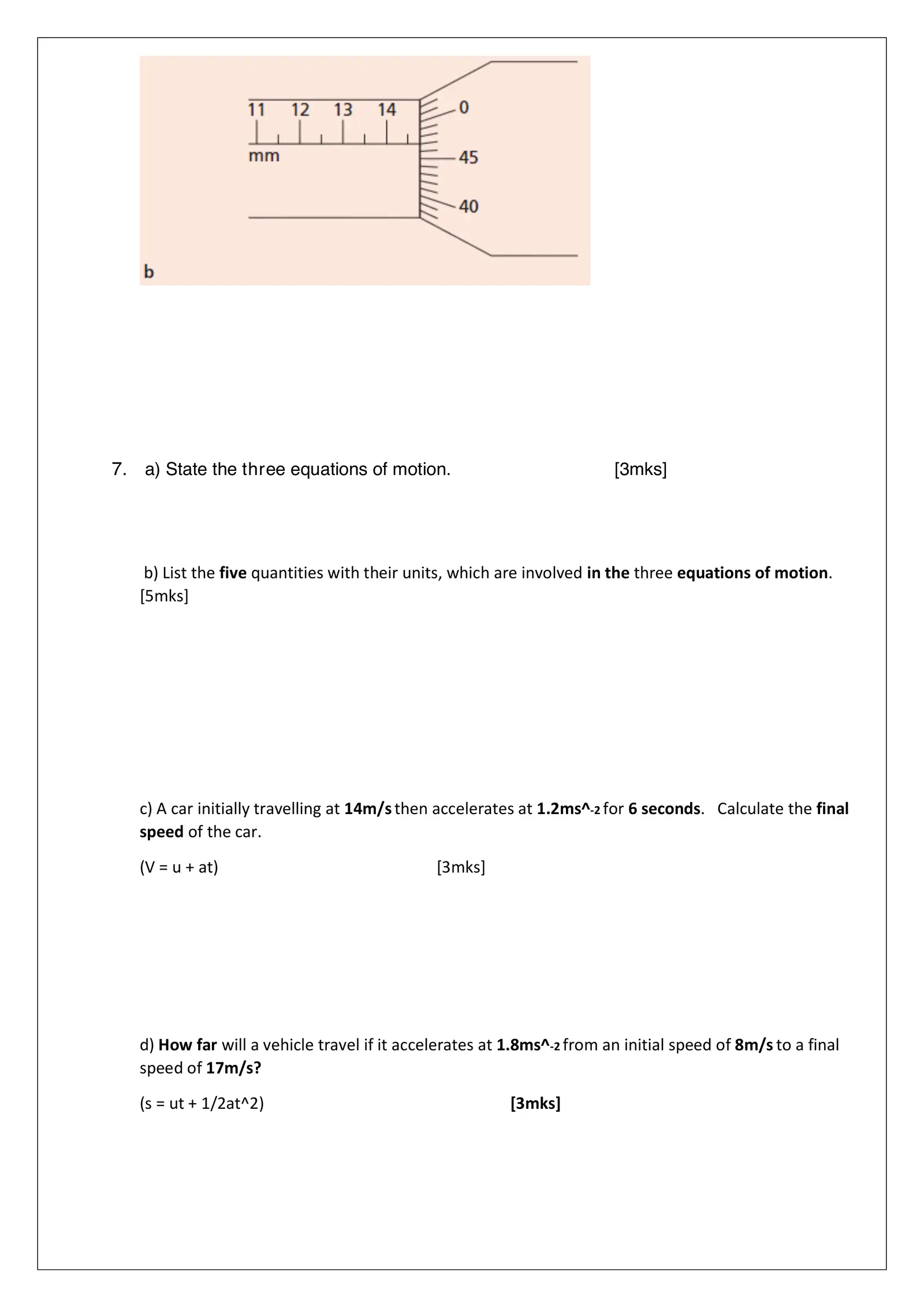7. a) State the three equations of motion. [3mks] b) List the five quantities with their units, which are involved in the three equations of motion. [5mks] c) A car initially travelling at 14m/s then accelerates at 1.2ms^-2 for 6 seconds. Calculate the final speed of the car. (V = u + at) [3mks] d) How far will a vehicle travel if it accelerates at 1.8ms^-2 from an initial speed of 8m/s to a final speed of 17m/s? (s = ut + 1/2at^2) [3mks]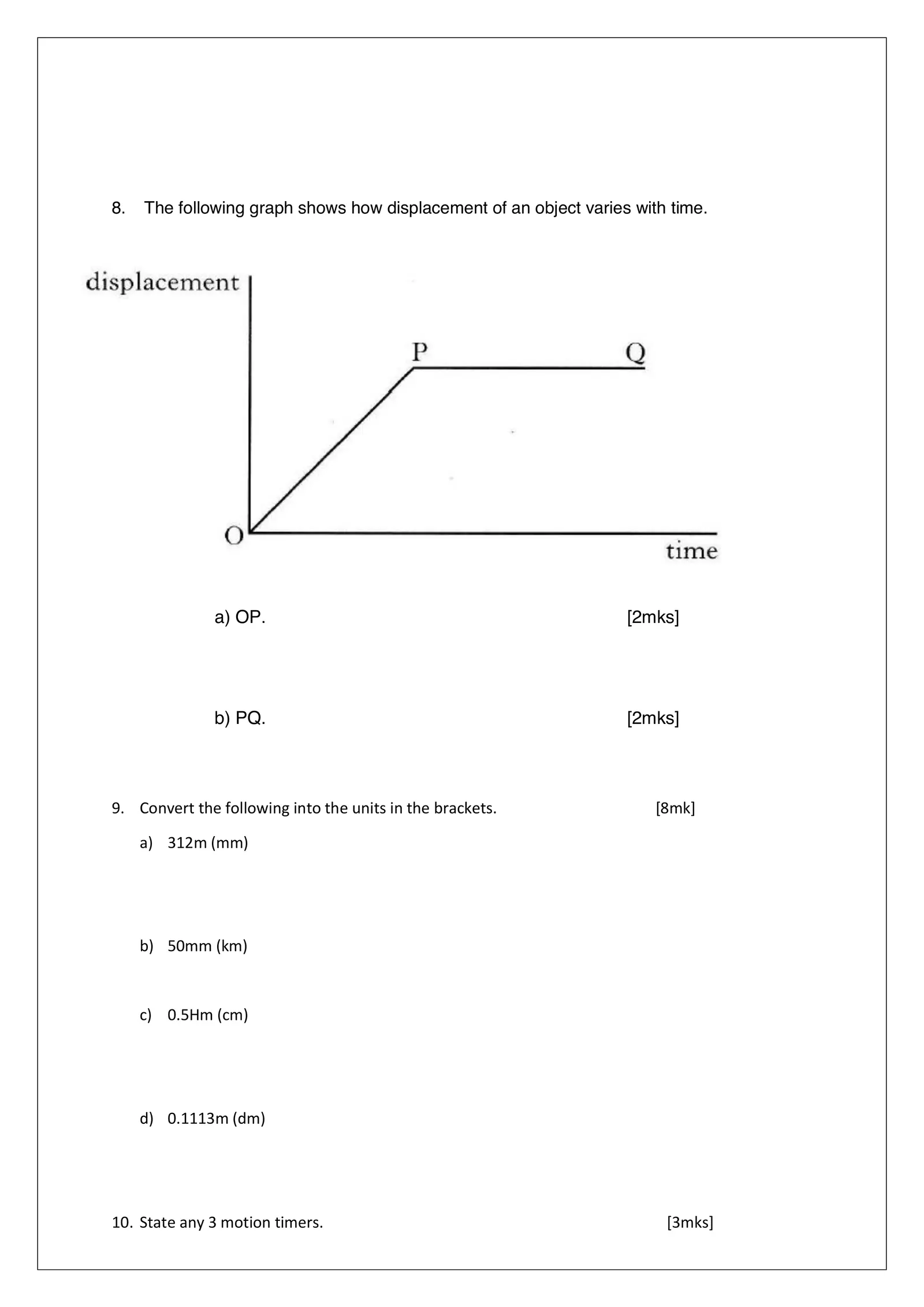8. The following graph shows how displacement of an object varies with time. a) OP. [2mks] b) PQ. [2mks] 9. Convert the following into the units in the brackets. [8mk] a) 312m (mm) b) 50mm (km) c) 0.5Hm (cm) d) 0.1113m (dm) 10. State any 3 motion timers. [3mks]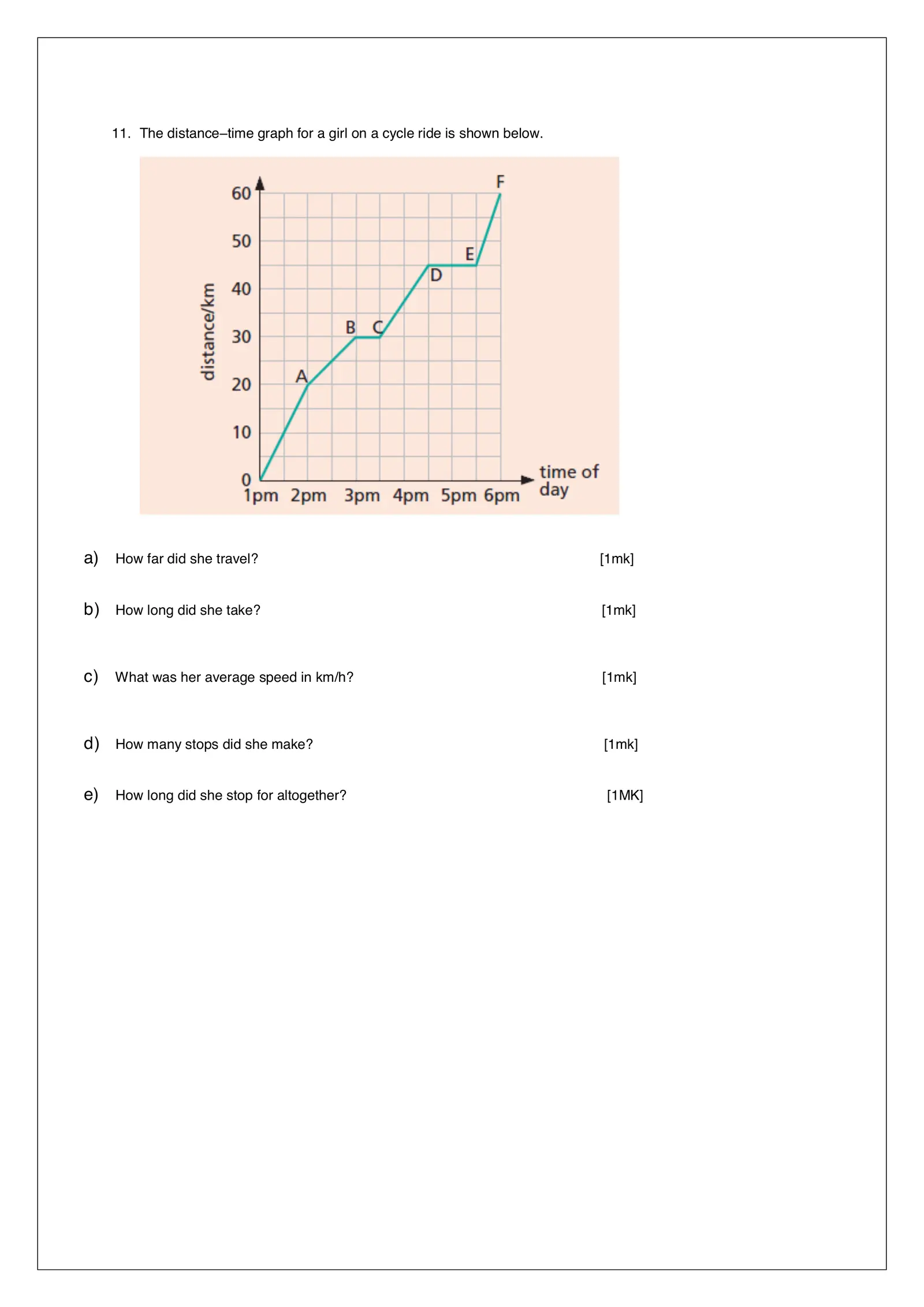11. The distance–time graph for a girl on a cycle ride is shown below. a) How far did she travel? [1mk] b) How long did she take? [1mk] c) What was her average speed in km/h? [1mk] d) How many stops did she make? [1mk] e) How long did she stop for altogether? [1MK]